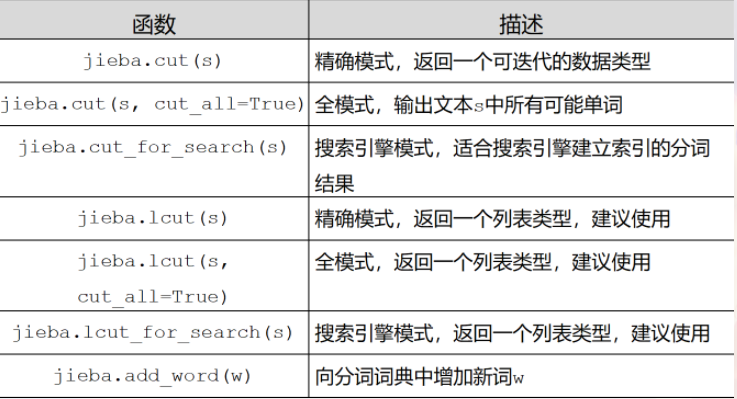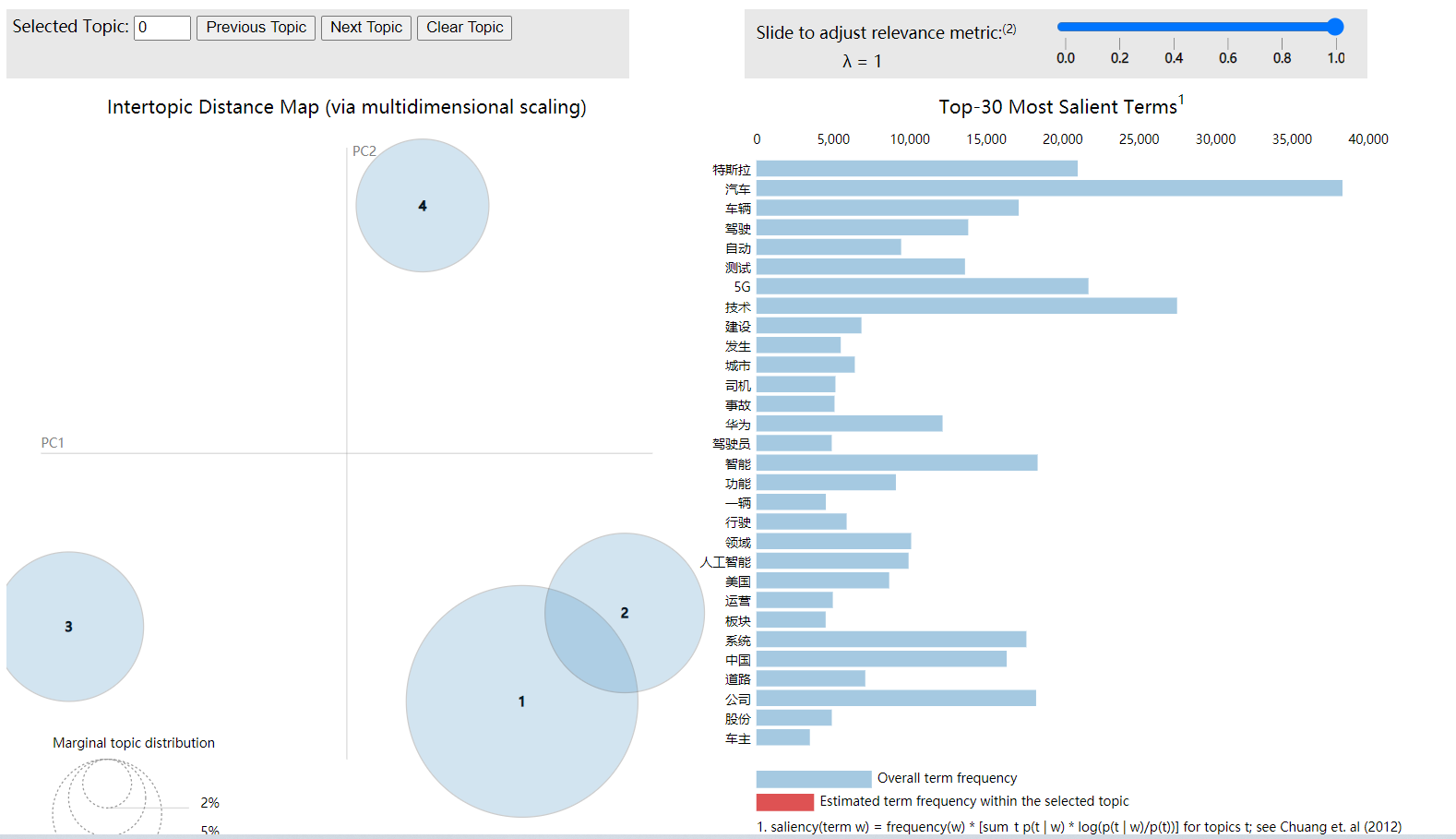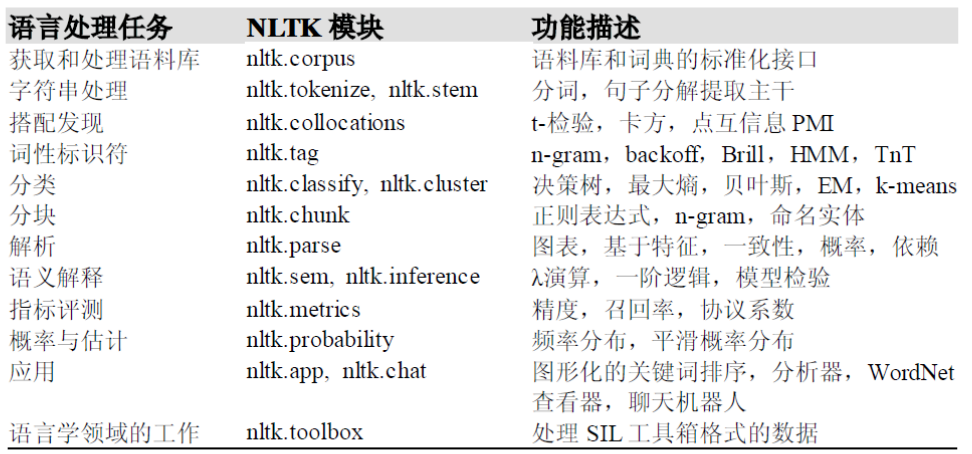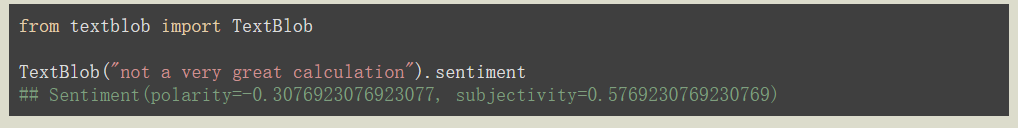# 前言

本文主要介绍python文本处理算法代码主要应用和一些基本原理

# 一、常用库📖

## 1. Jieba

jieba是支持中文分词的第三方库。

jieba库分词的三种模式：

1. 精准模式：把文本精准地分开，不存在冗余
2. 全模式：把文中所有可能的词语都扫描出来，存在冗余
3. 搜索引擎模式：在精准模式的基础上，再次对长词进行切分## 2. gensim

gensim 是一个通过衡量词组（或更高级结构，如整句或文档）模式来挖掘文档语义结构的工具

### 2.1. 构建词典（文集）

``from gensim import corporaimport jiebadocuments = ['工业互联网平台的核心技术是什么',            '工业现场生产过程优化场景有哪些']def word_cut(doc):    seg = [jieba.lcut(w) for w in doc]    return seg texts= word_cut(documents) '''为语料库中出现的所有单词分配了一个唯一的整数id'''dictionary = corpora.Dictionary(texts)dictionary.token2id# => {'互联网': 0,#     '什么': 1,#     '优化': 7,#     '哪些': 8,#     '场景': 9,#     '工业': 2,#     '平台': 3,#     '是': 4,#     '有': 10,#     '核心技术': 5,#     '现场': 11,#     '生产': 12,#     '的': 6,#     '过程': 13}``

### 2.2.  语料向量化

``'''该函数doc2bow()只计算每个不同单词的出现次数，将单词转换为整数单词id，并将结果作为稀疏向量返回'''bow_corpus = [dictionary.doc2bow(text) for text in texts]# => [[(0, 1), (1, 1), (2, 1), (3, 1), (4, 1), (5, 1), (6, 1)],# [(2, 1), (7, 1), (8, 1), (9, 1), (10, 1), (11, 1), (12, 1), (13, 1)]]``

### 2.3. 构建LDA模型

``from gensim.models.coherencemodel import CoherenceModelfrom gensim.models.ldamodel import LdaModel #分为10个主题ldamodel = LdaModel(corpus, num_topics=10, id2word = dictionary, passes=30,random_state = 1)ldamodel.print_topics(num_topics=num_topics, num_words=15)  #每个主题输出15个单词``

### 2.4. 评价LDA主题模型 （确认最优主题数）

``#计算困惑度def perplexity(num_topics):    ldamodel = LdaModel(corpus, num_topics=num_topics, id2word = dictionary, passes=30)    print(ldamodel.print_topics(num_topics=num_topics, num_words=15))    print(ldamodel.log_perplexity(corpus))    return ldamodel.log_perplexity(corpus) #计算coherencedef coherence(num_topics):    ldamodel = LdaModel(corpus, num_topics=num_topics, id2word = dictionary, passes=30,random_state = 1)    print(ldamodel.print_topics(num_topics=num_topics, num_words=10))    ldacm = CoherenceModel(model=ldamodel, texts=data_set, dictionary=dictionary, coherence='c_v')    print(ldacm.get_coherence())    return ldacm.get_coherence()``

``x = range(1,15)# z = [perplexity(i) for i in x]  #如果想用困惑度就选这个y = [coherence(i) for i in x]plt.plot(x, y)plt.xlabel('主题数目')plt.ylabel('coherence大小')plt.rcParams['font.sans-serif']=['SimHei']matplotlib.rcParams['axes.unicode_minus']=Falseplt.title('主题-coherence变化情况')plt.show()``

### 2.5. LDA模型结果进行可视化

``import pyLDAvis.gensimpyLDAvis.enable_notebook()data = pyLDAvis.gensim.prepare(lda, corpus, dictionary)pyLDAvis.save_html(data, 'E:/data/3topic.html')``## 3. ahocorasick (AC自动机)

ac自动机算法可以快速找到字符串里的字符段

ac自动机典型应用是用于统计和排序大量的字符串（但不仅限于字符串），所以经常被搜索引擎系统用于文本词频统计

``!pip install pyahocorasick  #安装import ahocorasick A = ahocorasick.Automaton() ''' 向trie树中添加单词 '''for index,word in enumerate("he her hers she".split()):    A.add_word(word, (index, word)) #检查trie树A.get("he")# (0,'he')A.get("cat","<not exists>")# '<not exists>'A.get("dog")# KeyError ''' 将trie树转化为Aho-Corasick自动机 '''A.make_automaton() ''' 找到所有匹配字符串， 没有匹配到则不执行 '''for item in A.iter("_hershe_"):    print item#(2,(0,'he'))#(3,(1,'her'))#(4, (2, 'hers'))#(6, (3, 'she'))#(6, (0, 'he'))``

## 4. SnowNLP

Snownlp是中文文本处理库，可以用于情感分析（朴素贝叶斯实现），文本分类

``from snownlp import SnowNLP s = SnowNLP(u'SnowNLP类似NLTK，是针对中文处理的一个Python工具库。')sentiments = s.sentimentsprint(sentiments)  #情感分数0~1``

## 5. NLTK

`Natural Language Toolkit`## 6. TextBlobtextblob的情感分析原理 – 知乎

# 二、算法原理

## 1. LDA主题模型

LDA模型认为主题可以由一个词汇分布来表示，而文章可以由主题分布来表示

## 2. AC自动机

AC自动机是KMP和trie的结合体。KMP算法适用于单模式串的匹配，而AC自动机适合多模式串的匹配。例如：在一篇文章中我们找一句话可以用KMP，找多句话适用于AC自动机。

## 3. TF-IDF

TF = (Number of times term T appears in the particular row) / (number of terms in that row)

IDF = log(N/n)

TF-IDF=TF`*`IDF

``tf1 = (train['tweet'][1:2]).apply(lambda x: pd.value_counts(x.split(" "))).sum(axis = 0).reset_index()  tf1.columns = ['words','tf']  for i,word in enumerate(tf1['words']):      tf1.loc[i, 'idf'] =np.log(train.shape/(len(train[train['tweet'].str.contains(word)]))) tf1['tfidf']=tf1['tf']*tf1['idf']``

## 4. LSI主题模型

LSI是最早出现的主题模型了，它的算法原理很简单，一次奇异值分解就可以得到主题模型，同时解决词义的问题，非常漂亮。但是LSI有很多不足，导致它在当前实际的主题模型中已基本不再使用。

1. SVD计算非常的耗时，尤其是我们的文本处理，词和文本数都是非常大的，对于这样的高维度矩阵做奇异值分解是非常难的。
2. 主题值的选取对结果的影响非常大，很难选择合适的k值。
3.  LSI得到的不是一个概率模型，缺乏统计基础，结果难以直观的解释。

LSI和LDA模型初试验 – tspeaking – 博客园

# 三、文本处理编码问题

## 1. chardet 库

chardet可以自动检查字符串编码类型

``import chardet str1 = 'hello'.encode('utf-8')  # encode 接受str,返回一个bytesprint(type(str1),str1) result = chardet.detect(str1)  # chardet 接受bytes类型,返回一个字典,返回内容为页面编码类型.print(type(result),result)codetype = result.get('encoding')print(codetype)  #>> <class 'bytes'> b'hello'#>> <class 'dict'> {'encoding': 'ascii', 'confidence': 1.0, 'language': ''}#>> ascii``

## 2. emoji 库

``import emojia = emoji.emojize(':grinning_face:', use_aliases=True)a = emoji.demojize('????', use_aliases=True)``

# References

LDA主题模型详解（面试的问题都在里面） – 知乎

LDA主题模型简介及Python实现_阿丢是丢心心的博客-CSDN博客_lda模型

SnowNLP，中文语言处理的必备工具 – 知乎